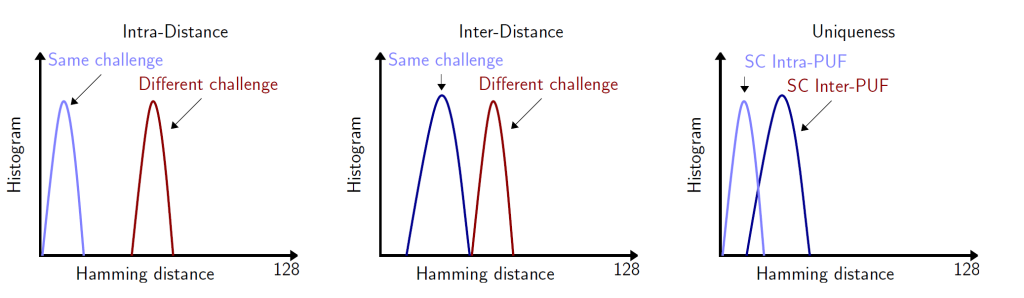Physical Unclonable Functions

Last quarter I took my first grad class at UCSB called CS:290T "Computational Algebra". It was a very eclectic class where different speakers would come to lecture us on some aspect of their research. Needless to say, there were many interesting topics brought forth that ranged from Boolean Functions (on which I wrote a paper and will post later), distributed security, error tolerant functions, LLL Algorithm, etc. Among all of those, there was one that intrigued me quite a lot: Physical Unclonable Functions. What got me, is that the concept is easy to understand and the implementation can be done with virtually anything you want. So without further ado, lets take a plunge into the fascinating world of PUFs.

1. Definition

Physical Unclonable Function (PUF) is a hardware implemented function in which the mapping between a challenge and its response is dependent on the hardware variations (eg. materials, electric params, design, etc.).

For example, your PUF could rely on the time (in milliseconds) it takes for electricity to go through a wire. Although the wire is cut similarly in all computers, the small fluctuations in length might be enough for two computers to get different results. Now the trick is to choose a physical property that would vary greatly from machine to machine, but within the same computer the result doesn't change too much.

2. Key Principles

• PUFi should be unique to each device i
• Each (xi,yi) pair should be independent and not linkable.

3. Usages

• Device Authentication
• Tamper Protection (e.g: Anti-Counterfeit, Intellectual Property Protection, etc.)
• Cryptography (e.g: Key generation)

4. Types of PUF

You can get pretty creative with what you want to use as a basis for your PUF. (I've seen some cool ideas such as camera CCDs or magstripes). However, the most typical types of PUF are:

• SRAM PUF
• These use the SRAM memory on board.
• Delay PUF
• These use the variation in delays of wires and gates on silicon wafers.
• Some of these implementations are: Ring Oscillator, Arbiter, Multiplexor-based
• Butterfly PUF
• These are similar to the SRAM PUF but can be impmented on SRAM FPGAs because they are implemented by cross-coupling two latches or flip-flops.
• Optical PUFs, Metal Resistance PUF, and so many more...

5. Properties of a PUF

• Steadiness: A PUF that always generate the same response even when varying operating conditions (e.g: supply voltage, and temperature) is said to be steady. 
• Randomness: The measure of bias among all challenges on all PUF instances. 
• Diffuseness: The degree of difference (Hamming distance) among different responses to different challenges for the same PUF. (The expected diffuseness is 50%). 
• Uniqueness: Reliability measures the consistency with which a given PUF reproduces a response to repeated application of a given challenge. 

6. PUF Mathematical Notation

• Creation of a PUF Instance: $P \equiv \{ \text{puf}_{i} \leftarrow P.\text{Create}(r_{i}^{C}): \forall{i,r_{i}^{C}} \xleftarrow{\}\{0,1\}^{*} \}$
• Evaluation of a PUF Instance: $y_{i}^{(j)}(x) \leftarrow \text{puf}_{i}(x).\text{Eval}(r_{j}^{E} \xleftarrow{\}\{0,1\}^{*})$
• $r^{C}$ and $r^{E}$ represent randomized fair coin tosses.

7. Measuring PUFs

There are two basic measurements that we will use to calculate the properties of a PUF.

• Intra-Distance refers to the distance between two responses from the same PUF instance using the same challenge.
• $D_{\text{puf}_{i}}^{intra}(x) \triangleq{\delta} \text{dist}[Y_{i}(x);Y_{i}^{'}(x)]$
• $Y_{i}(x)$ and $Y_{i}^{'}(x)$ refer to distinct random evaluations of the same pufand dist[_;_] denotes the hamming distance between two random variables.
• Intra-distance will give us an idea of how reproducible or reliable this given PUF is. Remember that we want to find a PUF that is consistent within the same device.
• Inter-Distance refers to the distance between two responses from different PUF instances using the same challenge.
• $D_{\text{puf}_{i}}^{inter}(x) \triangleq{\delta} \text{dist}[Y(x);W(x)]$
• $Y(x)$ and $W(x)$ refer to distinct random evaluations of the  two pufs.
• Inter-distance will give us an idea of how distinct this PUF will be across multiple devices. Remember that we want this function to be as different as possible across multiple devices.
• For more in depth calculations check out this link.Quantative tests yield to model a PUF by Hori et al., Quantitative and Statistical Performance Evaluation of Arbiter Physical Unclonable Functions on FPGAs. ReConFig2010, pp.298-303, 2010.